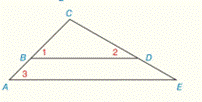Chapter 2.CR, Problem 34CR### Elementary Geometry for College St...

6th Edition
Daniel C. Alexander + 1 other
ISBN: 9781285195698

#### Solutions

Chapter
Section### Elementary Geometry for College St...

6th Edition
Daniel C. Alexander + 1 other
ISBN: 9781285195698
Textbook Problem
3 views

# Given: ∠ 1 is complementary to ∠ 2 ; ∠ 2 is complementary to ∠ 3 Prove: B D ¯ ≅ A E ¯To determine

To check:

The given statement.

Explanation

Given:

1 is complementary to 2 and 2 is complementary to 3

Figure (1)

Properties:

(1) If two lines are cut by a transversal so that corresponding angles are congruent, then these lines are parallel.

(2) Complementary angles add up to 90°

Approach:

1 is complementary to 2.

m1+m2=90°(1)

2 is complementary to 3.

m2+m3=90°(2)

Subtract equation (2) from equation (1).

m1+m2m2m3=90°90°m1m3=0m1=m313

1 and 3 are corresponding congruent angles.

So,

BD¯AE¯.

The proof for the given statement is shown in the following table,

 PROOF Statements Reasons 1. ∠1 is complementary to ∠2 1

### Still sussing out bartleby?

Check out a sample textbook solution.

See a sample solution

#### The Solution to Your Study Problems

Bartleby provides explanations to thousands of textbook problems written by our experts, many with advanced degrees!

Get Started

#### Write the sum in expanded form. 9. j=0n1(1)j

Single Variable Calculus: Early Transcendentals, Volume I

#### In Exercise 1-6, express each equation in logarithmic form. 323/5=8

Finite Mathematics for the Managerial, Life, and Social Sciences

#### True or False: If converges absolutely, then it converges conditionally.

Study Guide for Stewart's Single Variable Calculus: Early Transcendentals, 8th